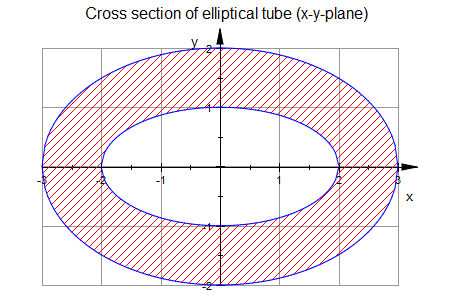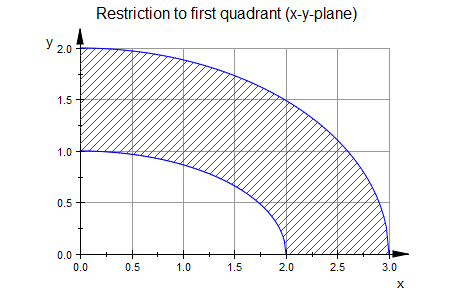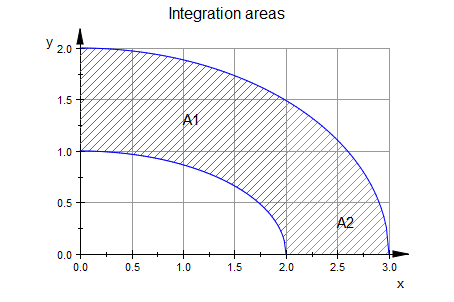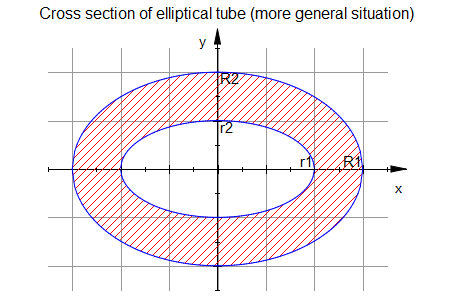# Using Symbolic Math Toolbox to Compute Area Moments of Inertia

Once more I am pleased to introduce guest blogger Kai Gehrs. Kai has been a Software Engineer at MathWorks for the past five years working on the Symbolic Math Toolbox. He has a background in mathematics and computer science. He already contributed to my BLOG in the past writing about Using Symbolic Equations And Symbolic Functions In MATLAB as well as on approaches for Simplifying Symbolic Results.

### Contents

If you are interested in using MATLAB and the Symbolic Math Toolbox in teaching some basics in mechanical engineering, this might be of interest to you.

Computing area moments of inertia is an important task in mechanics. For example, area moments of inertia play a critical role in stress, dynamic, and stability analysis of structures. In this article, we use capabilities of the Symbolic Math Toolbox to compute area moments for cross sections of elliptical tubes.

We start with a basic case involving only numeric parameters, and then make the computations more general by introducing symbolic parameters.

All plots used in this article have been created in the MuPAD Notebook app. You can find the source code for these plots at the end of the article.

#### Basic Example: Cross Section of an Elliptical Tube

The following picture shows the cross section of an elliptical tube.The following ellipses define outer and inner contours of the section:

• $y = \pm 2 \, \sqrt{1 - \frac{x^2}{9}}$ describe the outer contour line for $x \in [-3,3]$.
• $y = \pm \sqrt{1 - \frac{x^2}{4}}$ describe the inner contour line for $x \in [-2,2]$.

We will compute the area moment of inertia of this section with respect to the $x$-axis.

#### Area Moment Of Inertia

The moment of inertia of an area $A$ with respect to the $x$-axis is defined in terms of the double integral

$$I_x = {\int \int}_A y^2\, \mathrm{d}A.$$

You can find this definition on Wikipedia.

#### Math Behind This Example

In our example, the area $A$ is the hatched area shown in the previous plot. Taking advantage of the symmetry of the section with respect to both the $x$- and $y$-axes, we can restrict our computations to the first quadrant of the $x-y$-plane.To compute the necessary double integral over the hatched area, we divide this area into two separate areas $A1$ and $A2$.To compute the final area moment of inertia about the x-axis, we multiply the sum of the double integrals over $A_1$ and $A_2$ by four.

$$I_x = {\int\int}_A y^2 \, \mathrm dA = 4 \cdot \Big({\int\int}_{A_1} y^2 \, \mathrm dA_1 + {\int\int}_{A_2} y^2 \, \mathrm dA_2\Big).$$

Now, we only need to compute these two double integrals. The mathematical theory behind multi-dimensional integration tells us that we can rewrite each of these double integrals in terms of two integrals:

$$I_1 = {\int\int}_{A_1} y^2 \, \mathrm dA_1 = \int_0^2 \int_{\sqrt{1 - \frac{x^2}{4}}}^{2 \, \sqrt{1 - \frac{x^2}{9}}} y^2 \, \mathrm dy \, \mathrm dx,$$

$$I_2 = {\int\int}_{A_2} y^2 \, \mathrm dA_2 = \int_2^3 \int_{0}^{2 \, \sqrt{1 - \frac{x^2}{9}}} y^2\, \mathrm dy\, \mathrm dx.$$

The int function from the Symbolic Math Toolbox can compute these integrals.

#### Symbolic Integration

We define $x$ and $y$ as symbolic variables:

syms x y;


We start computing the inner integral $\int_{\sqrt{1 - \frac{x^2}{4}}}^{2 \, \sqrt{1 - \frac{x^2}{9}}} y^2 \, \mathrm dy$ of $I_1$:

innerI1 = int(y^2, y, sqrt(1-x^2/4), 2*sqrt(1-x^2/9))

innerI1 =
(8*(9 - x^2)^(3/2))/81 - (4 - x^2)^(3/2)/24


Next, we integrate innerI1 with respect to $x$ from $0$ to $2$. This provides $I_1$:

I1 = int(innerI1, x, 0, 2)

I1 =
3*asin(2/3) - pi/8 + (74*5^(1/2))/81


We use the same strategy to compute $I_2$. First, we compute the inner integral $\int_{0}^{2 \, \sqrt{1 - \frac{x^2}{9}}} y^2\, \mathrm dy$. Then, we integrate the resulting expression with respect to $x$ from $2$ to $3$:

I2 = int(int(y^2, y, 0, 2*sqrt(1-x^2/9)), x, 2, 3);


Hence, the area moment of inertia of the elliptical tube with respect to the $x$-axis is

Ix = 4 * (I1 + I2)

Ix =
(11*pi)/2


We can approximate the result numerically by using the vpa function. For example, we approximate the result using 5 significant (nonzero) digits:

vpa(Ix, 5)

ans =
17.279


#### Advanced Example: Cross Section of an Elliptical Tube Defined by Symbolic Parameters

We can use a numerical integrator, such as MATLAB's integral2, to compute the area moment of inertia in the previous example. A numerical integrator might return slightly less accurate results, but other than that there is not much benefit from using symbolic integration there.

But what if we consider the cross section of a more general elliptical tube whose shape is defined by arbitrary symbolic parameters?

For example, we want to compute the area moment of inertia of this elliptical tube.Its contour lines are still ellipses, but they are defined by the symbolic parameters $r_1$, $r_2$, $R_1$ and $R_2$:

• $y = \pm R_2 \, \sqrt{1 - \frac{x^2}{R_1^2}}$ describe the outer contour line for $x \in [-R_1,R_1]$.
• $y = \pm r_2 \, \sqrt{1 - \frac{x^2}{r_1^2}}$ describe the inner contour line for $x \in [-r_1,r_1]$.

Now we need to compute these integrals:

$$I_1 = {\int\int}_{A_1} y^2 \, \mathrm dA_1 = \int_0^{r_1} \int_{r_2 \, \sqrt{1 - \frac{x^2}{r_1^2}}}^{R_2 \, \sqrt{1 - \frac{x^2}{R_1^2}}} y^2 \, \mathrm dy \, \mathrm dx,$$

$$I_2 = {\int\int}_{A_2} y^2 \, \mathrm dA_2 = \int_{r_1}^{R_1} \int_{0}^{R_2 \, \sqrt{1 - \frac{x^2}{R_1^2}}} y^2\, \mathrm dy\, \mathrm dx.$$

Although the situation is more complicated now, we can still use the same strategy as above, using symbolic variables instead of numbers. Nevertheless, we need to add one more step: specify relationships between symbolic parameters. This is because the variables $r_1$, $r_2$, $R_1$, and $R_2$ can be any complex number, unless we explicitly restrict their values. For example, the system does not know if these variables are positive or negative, if $r_1 < R_1$ or otherwise. In this example, we want to specify that $r_1>0$, $r_2>0$, $R_1>r_1$, and $R_2>r_2$. In the Symbolic Math Toolbox, such restrictions are called assumptions on variables. They can be set by using the assume and assumeAlso functions:

syms x y r1 r2 R1 R2;
assume(r1 > 0);
assume(r2 > 0);
assumeAlso(r1 < R1);
assumeAlso(r2 < R2);
I1 = int(int(y^2, y, r2*sqrt(1-x^2/r1^2), R2*sqrt(1-x^2/R1^2)), x, 0, r1);
I2 = int(int(y^2, y, 0, R2*sqrt(1-x^2/R1^2)), x, r1, R1);
Ixgeneral = 4 * (I1 + I2);
pretty(Ixgeneral)


/          4     / r1 \ \         /                     4     / r1 \ \
|      3 R1  asin| -- | |         |             4   3 R1  asin| -- | |
3 |                \ R1 / |       3 |      3 pi R1              \ R1 / |
4 R2  | #1 + ---------------- |   4 R2  | #1 - -------- + ---------------- |
\             8         /         \         16             8         /
------------------------------- - ------------------------------------------
3                                      3
3 R1                                   3 R1

3
pi r1 r2
- ---------
4

where

/     2        3 \
| 5 R1  r1   r1  |    2     2 1/2
#1 == | -------- - --- | (R1  - r1 )
\    8        4  /


Now, let's substitute our symbolic parameters with the same values that we had in the first example. Do we get the same result? Let's double-check: when substituting $r_1=2,r_2,=1,R_1=3,R_2=2$ in Ixgeneral, do we get the same result for Ix as obtained for the section initially considered?

As expected, the general solution Ixgeneral reduces to the specific solution Ix when we substitute our original dimensions:

vpa(subs(Ixgeneral, [r1, R1, r2, R2],[2, 3, 1, 2]), 5)

ans =
17.279


Note that it is essential to set the assumptions on $r_1$, $r_2$, $R_1$ and $R_2$. By default, the Symbolic Math Toolbox assumes that all variables including symbolic parameters are arbitrary complex numbers. This makes computing integrals much more complicated and time-consuming. For example, try computing Ixgeneral without setting the assumptions on $r_1$, $r_2$, $R_1$ and $R_2$. In general, it can be impossible to even get the result without any additional assumptions.

To generate the graphics shown in this article, use MuPAD Notebook app. Note that the code in this section does not run in the MATLAB Command Window.

To open the MuPAD Notebook app:

1. On the MATLAB Toolstrip, click the Apps tab.
2. On the Apps tab, click the down arrow at the end of the Apps section.
3. Under Math, Statistics and Optimization, click the MuPAD Notebook button.

Alternatively, type mupad in the MATLAB Command Window.

Paste the following commands to a MuPAD notebook to obtain the four graphics used above:

Ellipse:= (x,a,b) -> sqrt(b^2*(1-x^2/a^2)):
f1 := plot::Function2d(Ellipse(x,2,1), x = -3..3):
f2 := plot::Function2d(Ellipse(x,3,2), x = -3..3):
f3 := plot::Function2d(Ellipse(x,3,2), x = -3..-2):
f4 := plot::Function2d(Ellipse(x,3,2), x = 2..3):
f5 := plot::Function2d(-Ellipse(x,2,1), x = -3..3):
f6 := plot::Function2d(-Ellipse(x,3,2), x = -3..3):
f7 := plot::Function2d(-Ellipse(x,3,2), x = -3..-2):
f8 := plot::Function2d(-Ellipse(x,3,2), x = 2..3):
f9 := plot::Function2d(Ellipse(x,2,1), x = 0..2, VerticalAsymptotesVisible = FALSE):
f10 := plot::Function2d(Ellipse(x,3,2), x = 0..3, VerticalAsymptotesVisible = FALSE):
f11 := plot::Function2d(Ellipse(x,3,2), x = 2..3, VerticalAsymptotesVisible = FALSE):
plot(plot::Hatch(f1,f2),plot::Hatch(f3),plot::Hatch(f4),f1,f2,
plot::Hatch(f5,f6),plot::Hatch(f7),plot::Hatch(f8),f5,f6,
Scaling = Constrained,
GridVisible = TRUE,
VerticalAsymptotesVisible = FALSE,
Height = 120, Width = 200,
Header = "Cross section of elliptical tube (x-y-plane)"):
plot(plot::Hatch(f9,f10),plot::Hatch(f11),f9,f10,
Scaling = Constrained,
GridVisible = TRUE,
Height = 120, Width = 200,
Header = "Restriction to first quadrant (x-y-plane)"):
plot(plot::Hatch(f9,f10,Color=RGB::Magenta),plot::Hatch(f11,Color = RGB::Green),f9,f10,
Scaling=Constrained,
GridVisible = TRUE,
plot::Text2d("A1",[1.0,1.25]),
plot::Text2d("A2",[2.5,0.25]),
Height = 120, Width = 200,
Header = "Integration areas"):
plot(plot::Hatch(f1,f2),plot::Hatch(f3),plot::Hatch(f4),f1,f2,
plot::Hatch(f5,f6),plot::Hatch(f7),plot::Hatch(f8),f5,f6,
plot::Text2d("r1",[1.8,0.05]),
plot::Text2d("r2",[0.05,0.85]),
plot::Text2d("R1",[2.74,0.05]),
plot::Text2d("R2",[0.05,1.84]),
Scaling = Constrained,
GridVisible = TRUE,
VerticalAsymptotesVisible = FALSE,
XTicksLabelsVisible = FALSE,
YTicksLabelsVisible = FALSE,
ViewingBox = [-3.5..3.5,-2.5..2.5],
Height = 120, Width = 200,
Header = "Cross section of elliptical tube (more general situation)"):

#### Have You Tried Symbolic Math Toolbox?

Have you used the Symbolic Math Toolbox in computations related to mechanics? Let me know here.

Published with MATLAB® R2013a

|